More

# Finding Horizontal Asymptotes of Rational Functions

Remember that an asymptote is a line that the graph of a function approaches but never touches. Rational functions contain asymptotes, as seen in this example: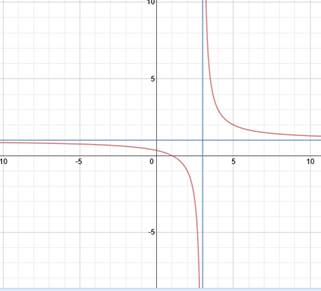In this example, there is a vertical asymptote at x = 3 and a horizontal asymptote at y = 1. The curves approach these asymptotes but never cross them.

The method used to find the horizontal asymptote changes depending on how the degrees of the polynomials in the numerator and denominator of the function compare.

• If both polynomials are the same degree, divide the coefficients of the highest degree terms.

Example: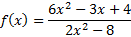Both polynomials are 2nd degree, so the asymptote is at• If the polynomial in the numerator is a lower degree than the denominator, the x-axis (y = 0) is the horizontal asymptote.
• If the polynomial in the numerator is a higher degree than the denominator, there is no horizontal asymptote. There is a slant asymptote, which we will study in a later lesson.

Examples: Find the horizontal asymptote of each rational function:First we must compare the degrees of the polynomials. Both the numerator and denominator are 2nd degree polynomials. Since they are the same degree, we must divide the coefficients of the highest terms.

In the numerator, the coefficient of the highest term is 4.

In the denominator, the coefficient of the highest term is an understood 1.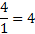The horizontal asymptote is at y = 4.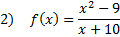First we must compare the degrees of the polynomials. The numerator contains a 2nd degree polynomial while the denominator contains a 1st degree polynomial.

Since the polynomial in the numerator is a higher degree than the denominator, there is no horizontal asymptote. There is a slant asymptote instead.First we must compare the degrees of the polynomials. The numerator contains a 1st degree polynomial while the denominator contains a 3rd degree polynomial.

Since the polynomial in the numerator is a lower degree than the denominator, the horizontal asymptote is located at y=0.

Practice: Find the horizontal asymptote of each rational function.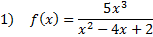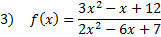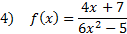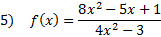Answers: 1) None 2) y = 7 3)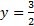4) y = 0 5) y = 2